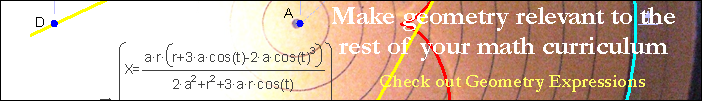FormulaeArea of ParallelogramSurface Area of Rectangular PrismVolume of SphereSurface Area of SphereLaw of SinesPythagorean TheoremSegment Area in DegreesThe area of a segment is equal to the area of the sector reduced by the area of the triangle formed by the chord and two radii. It is convenient to use the triangle area formula A = 1/2 ab sin C, which becomes the form seen here.Segment Area in RadiansThe area of a segment of a circle is the sector area decreased by the area of the triangle formed by the chord and two radii. The triangle area formula A = 1/2 a b sin C is convenient, becoming the formula seen here.